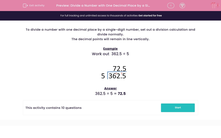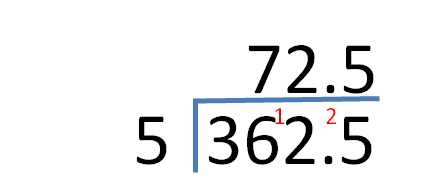# Divide a Number with One Decimal Place by a Single-Digit Number

In this worksheet, students divide numbers with one decimal place by a single-digit number.Key stage:  KS 2

Curriculum topic:   Maths and Numerical Reasoning

Curriculum subtopic:   Decimals

Difficulty level:#### Worksheet Overview

To divide a number with one decimal place by a single-digit number, set out a division calculation and divide normally.

The decimal points will remain in line vertically.

Example

Work out  362.5 ÷ 5